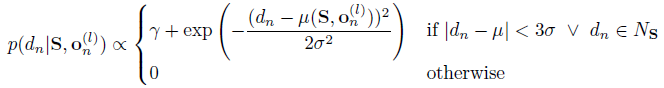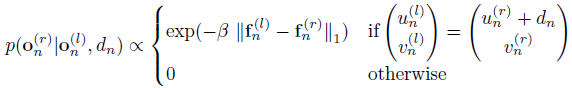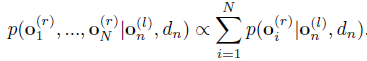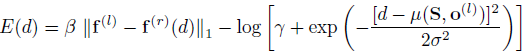# 文摘：高效大规模立体匹配

Geiger.A, Efficient large-scale stereo matching, In ACCV(2010)

1. 引言

2.相关工作

3.ELAS匹配

3.1 支撑点

3.2 立体匹配的生成模型

(dn on(l)on(r) ,S )  ∝  p(dn Son(l)p(on(r) |on(l)dn)µ(on(l)) = aunbi vci1. 已知 和 on(l)，由p(dn | Son(l)得到深度 dn

2. 已知on(l) 和 dn，由p(on(r) on(l)dn)得到观察 on(r)

3.3 视差估计

dn* = argmax p(dn |on(l),o1(r), ... ,oN(r),  S)

p(dn | on(l)o1(r), ... , oN(r),  S p(dn |S,on(l))p(o1(r), ... , oN(r) | on(l),dn)4. 实验评估

5. 结论和展望